# 7.6 Tuning the Wrapping Behavior

Wrappable segments are implemented internally using artificial linear elastic forces to draw the knots together and keep them from penetrating obstacles. These artificial forces are invisible to the simulation: the wrapping segment has no mass, and the knot forces are used to create what is essentially a first order physics that “shrink wraps” each segment around the obstacles at the beginning of each simulation step, forming a shortest-distance geodesic curve from which the wrapping contact points A and B are calculated. This process is now described in more detail.

Assume that a wrappable segment has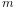knots, indexed by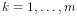, each located at a position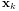. Two types of artificial forces then act on each knot: a wrapping force that pulls it closer to other knots, and contact forces that push it away from wrappable obstacles. The wrapping force is given by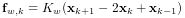where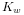is the wrapping stiffness. To determine the contact forces, we compute, for each wrappable, the knot’s distance to the surface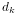and associated normal direction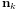, where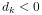implies that the knot is inside. These quantities are determined either analytically (for analytic wrappables, Table 7.1), or using a signed distance grid (for general wrappables, Section 7.3). The contact forces are then given by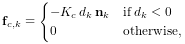where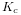is the contact stiffness.

The total force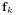acting on each knot is then given by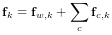where the latter term is the sum of contact forces for all wrappables. If we let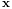and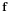denote the aggregate position and force vectors for all knots, then computing the wrap path involves finding the equilibrium position such that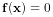. This is done at the beginning of each simulation step, or whenever updateWrapSegments() is called, and is achieved iteratively using Newton’s method. If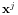and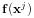denote the positions and forces at iteration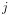, and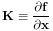denotes the local force derivative (or “stiffness”), then the basic Newton update is given by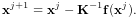In practice, to help deal with the nonlinearities associated with contact, we use a damped Newton update,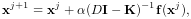(7.1)

where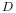is a constant wrap damping parameter, and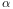is an adaptively computed step size adjustment. The computation of (7.1) can be performed quickly, in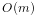time, since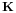is a block-tridiagonal matrix, and the number of iterations required is typically small (on the order of 10 or less), particularly since the iterative procedure continues across simulation steps and so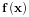does not need to be brought to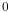for any given step. The maximum number of Newton iterations used for each time step is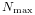.

Again, it is important to understand the artificial knot forcesdescribed here are separate from the physical spring/muscle tension forces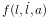discussed in Sections 3.1.1 and 4.4.1, and only facilitate the computation of each wrappable segment’s path around obstacles.

The default values for the wrapping parameters are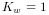,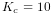,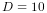, and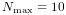, and these often give satisfactory results without the need for modification. However, in some situations the default muscle wrapping may not perform adequately and it is necessary to adjust these parameters. Problems may include:

• The wrapping path does not settle down and tends to “jump around”. Solutions include increasing the damping parameteror the maximum number of wrap iterations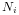. For general wrapping surfaces (Section 7.3), one should also ensure that the surface is sufficiently smooth.

• A wrapping surface is too thin and so the wrapping path “jumps through” it. Solutions include increasing the damping parameter, increasing the number of knots in the segment, or decreasing the simulation step size. An alternative approach is to use an alternative wrapping surface (Section 7.5) that is thicker and better behaved.

Wrapping parameters are exported as properties of MultiPointSpring and MultiPointMuscle, and may be changed in code (using their set/get accessors), or interactively, either by exposing them through a control panel, or by selecting the spring/muscle in the GUI and choosing Edit properties ... from the right-click context menu. Property values include:

wrapStiffness

Wrapping stiffnessbetween knot points (default value 1). Since the wrapping behavior is determined by the damping to stiffness ratio, it is generally not necessary to change this value.

wrapDamping

Damping factor(default value 10). Increasing this value relative toresults in wrap path motions that are smoother and less likely to penetrate obstacles, but which are also less dynamically responsive. Applications generally work with damping values between 10 and 100 (assuming).

contactStiffness

Contact stiffnessused to resolve obstacle penetration (default value 10). It is generally not necessary to change this value. Decreasing it will increase the distance that knots are permitted to penetrate obstacles, which may result in a slightly more stable contact behavior.

maxWrapIterations

Maximum number of Newton iterationsper time step (default value 10). If the wrapping simulation exhibits instability, particularly with regard to obstacle contact, increasing the number of iterations (to say 100) may help.

In addition, MultiPointSpring and MultiPointMuscle also export the following properties to control the rendering of knot and A/B points:

drawKnots

If true, renders the knot points in each wrappable segment. This can be useful to visualize the knot density. Knots are rendered using the style, size, and color given by the pointStyle, pointRadius, pointSize, and pointColor values of the spring/muscle’s render properties.

drawABPoints

If true, renders the A/B points. These are the first and last points of contact that a wrap segment makes with each wrappable, and correspond to the points where the spring/muscle’s tension acts on that wrappable (Section 7 and Figure 7.4). A/B points are rendered using the style and size given by the pointStyle, pointRadius (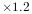) and pointSize values of the spring/muscle’s render properties, and the color given by the ABPointColor property.